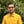Trusted answers to developer questions
Trusted Answers to Developer Questions

Related Tags

d
atanh
math
communitycreator

# What is the atanh() function in D?Harris Amjad

Grokking Modern System Design Interview for Engineers & Managers

Ace your System Design Interview and take your career to the next level. Learn to handle the design of applications like Netflix, Quora, Facebook, Uber, and many more in a 45-min interview. Learn the RESHADED framework for architecting web-scale applications by determining requirements, constraints, and assumptions before diving into a step-by-step design process.

## Overview

The atanh() function returns the inverse hyperbolic tangent of a number.

The equation below shows the mathematical representation of the atanh() function:

$atanh(x)=\frac {1}{2}ln(\frac {1+x}{1-x})$

Note: std.math is required for this function.

### Syntax

atanh(number)
//number can be real, float, or double.


### Parameter

This function requires a number as a parameter.

Note: The parameter should be between -1 and 1.

### Return value

The atanh() function returns the inverse hyperbolic tangent of a number that is sent as a parameter.

### Example

The code below shows the use of the atanh() function in D.

import core.stdc.stdio;import std.stdio;//header required for functionimport std.math;int main() {    //positive number    writeln("The value of atanh(0.5) :", atanh(0.5));    // negative number    writeln("The value of atanh(-0.5) :", atanh(-0.5));    //-1    writeln("The value of atanh(-1.0): ",atanh(-1.0) );    //1    writeln("The value of atanh(1.0): ",atanh(1.0) );    // >1    writeln("The value of atanh(2.0): ",atanh(2.0) );    // <-1    writeln("The value of atanh(-2.0): ",atanh(-2.0) );    return 0;}

### Explanation

• Line 4: We add the std.math header required for atanh() function.
• Line 8: We use atanh() to calculate the inverse hyperbolic tangent of the positive number (-1< number <1).
• Line 11: We use atanh() to calculate the inverse hyperbolic tangent of the negative number (-1< number <1).
• Line 14: We use atanh() to calculate the inverse hyperbolic tangent of -1.
• Line 17: We use atanh() to calculate the inverse hyperbolic tangent of 1.
• Line 20: We use atanh() to calculate the inverse hyperbolic tangent of number>1.
• Line 23: We use atanh() to inverse the hyperbolic tangent of number<-1.

RELATED TAGS

d
atanh
math
communitycreator

CONTRIBUTORHarris Amjad

Grokking Modern System Design Interview for Engineers & Managers

Ace your System Design Interview and take your career to the next level. Learn to handle the design of applications like Netflix, Quora, Facebook, Uber, and many more in a 45-min interview. Learn the RESHADED framework for architecting web-scale applications by determining requirements, constraints, and assumptions before diving into a step-by-step design process.

Keep Exploring

Learn in-demand tech skills in half the time